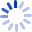# Ultimate Cheat Sheet for College Math: Algebra - Trig - Calculus - Linear Algebra - Diff Eq.

Everything a college student needs from algebra, trigonometry, precalculus, calculus, linear algebra, differential equations, and physics.• Book Details
• Seller